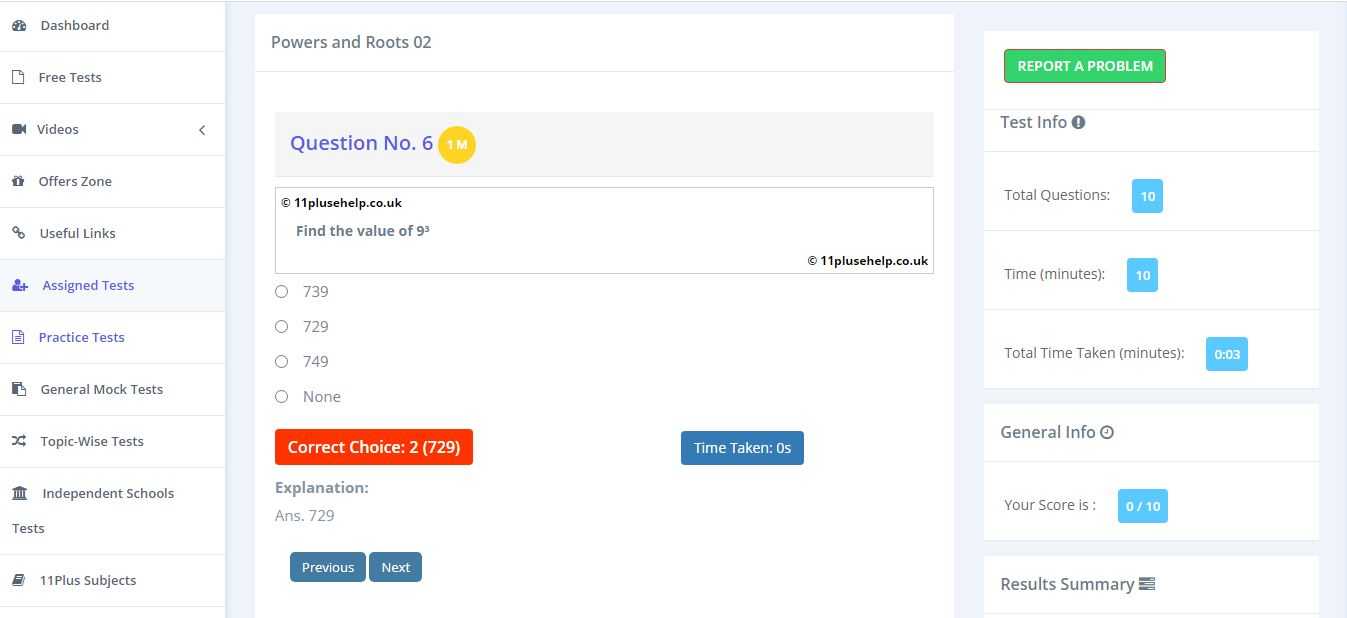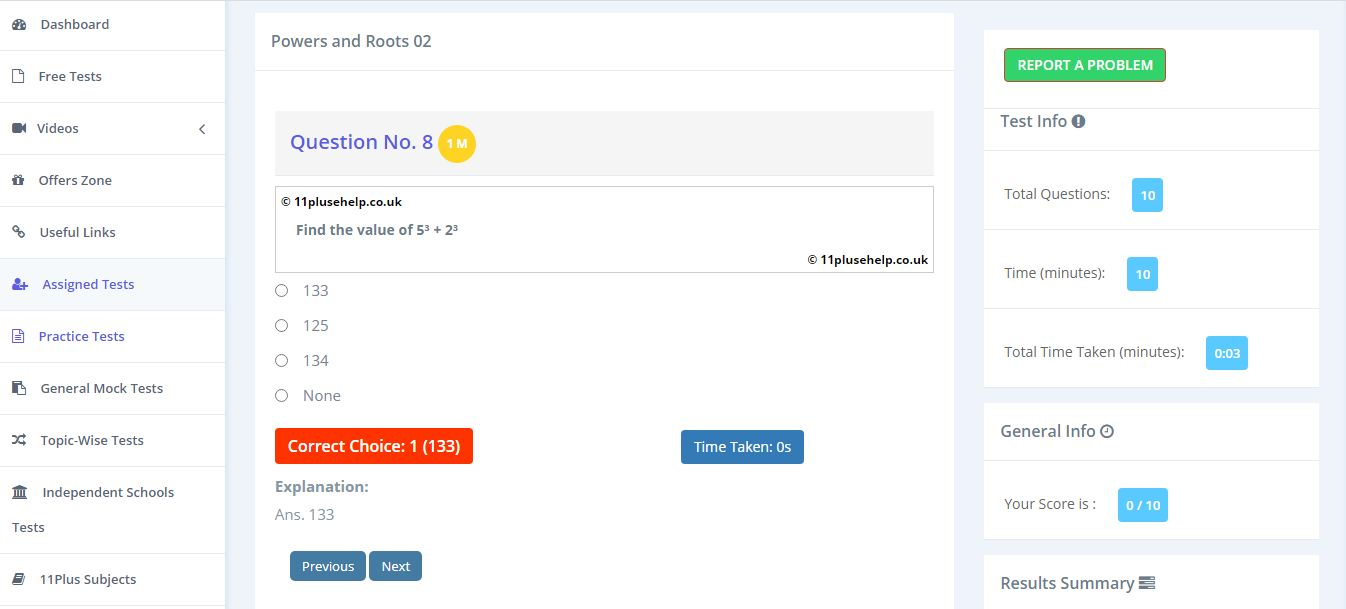Latest Results:

11 Plus Powers and roots

11PluseHelp.co.uk provides a plethora of practice papers on 11 Plus Powers and roots.

Powers :

If any number is represented in the form of ab, then a is called exponent and b is called power.

ab = a raised to power b = a x a x a - - - - - b times i.e., a is written b times.

let us understand with one example,

4 x 4 x 4 x 4 x 4 x 4 = 46

37 = 3 x 3 x 3 x 3 x 3 x 3 x 3 = 2187

* a0 = 1

The root of a number :

The root of a number 'a' is a number, which, when multiplied by itself a given number of times, equals x.

Let us understand with examples,

The square root of 9 is 3 because '3' is multiplied twice the product is 9.

3 x 3 = 9

The square root of 9 is represented by

The cube root of 8 is 2 because '2' is multiplied thrice the product is 8.

2 x 2 x 2 = 8

The cube root of 8 is represented by

Some examples from our 11 Plus Practice PapersYou can access 11 plus maths papers on different topics here:

https://www.11plusehelp.co.uk/11-plus-maths-practice-tests

11PluseHelp.co.uk is a one-stop shop for 11 Plus exam preparation, including 11 Plus Independent schools preparation.

We focus on fundamentals, logic, basics and cover most of the syllabus for all 11 Plus exam patterns in the UK. For example, We cover Maths topic wise questions and fundamentals and how to apply them. We have been continuously adding 11 plus practice papers regularly.

We have loads of questions useful for the preparation of CEM, CSSE and GL assessment and any other 11 Plus entrance exams in the UK. Children will love to take these tests, and learning is fun at 11plusehelp.co.uk.

At 11plusehelp.co.uk/, we possess the following resources:

Maths- 11 Plus Maths Worksheets, 11 Plus Maths Papers free download, 11 Plus Maths topics pdfs.

English- 11 Plus English papers with answers, 11 Plus comprehension with answers, 11 English comprehensions multiple-choice, English reasoning test.

Verbal and Non-Verbal Reasoning- 11 Plus Verbal Reasoning worksheets free, Verbal and Non-Verbal Reasoning tests, easy Non-Verbal Reasoning Worksheets

Practice Tests- Eleven plus sample papers, free 11 plus papers with answers, 11 plus mock test papers pdf, 11 exam question and answers, UK 11 plus exam questions, 11 online tests in Maths and English with 11 Plus answers.

You can access 11 Plus FREE Papers by visiting the below link:
https://www.11plusehelp.co.uk/11-plus-free-online-papers

You can access 11 Plus FREE Sample Papers by visiting the below link:
https://www.11plusehelp.co.uk/11-plus-sample-papers

11 Plus complete solution features:

https://11plusehelp.co.uk/blog/2017/05/01/11-plus-complete-solution/

Practice and Perseverance Over Genius and Talent

Thanks,

11Plusehelp.co.uk